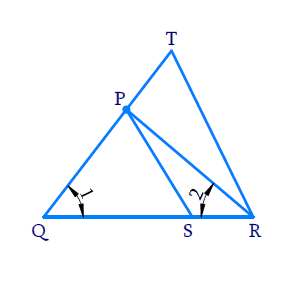# Ex.6.3 Q4 Triangles Solution - NCERT Maths Class 10

Go back to  'Ex.6.3'

## Question

In Figure \begin{align}\frac{QR}{QS}=\frac{QT}{PR}\end{align} and $$\angle 1 = \angle 2$$. Show that $$\Delta PQS\sim{\ }\Delta TQR$$ .

DiagramVideo Solution
Triangles
Ex 6.3 | Question 4

## Text Solution

Reasoning:

As we know if one angle of a triangle is equal to one angle of the other triangle and the sides including these angles are proportional, then the two triangles are similar.

This criterion is referred to as the SAS (Side–Angle–Side) similarity criterion for two triangles.

Steps:

In $$\triangle PQR$$

\begin{align} \angle 1&=\angle 2\\ \Rightarrow \quad PR &= PQ \end{align}

(In a triangle sides opposite to equal angles are equal)

In $$\Delta PQR$$ and $$\Delta TQR$$

\begin{align} & \angle PQS=\angle TQR=\angle 1\\&(\text{Same angle}) \\\\ & \frac{QR}{QS}=\frac{QT}{PQ}\quad\left( \because \ {PR =PQ} \right) \\ & \Rightarrow \Delta PQR-\Delta TQR\\&( \because \text{ SAS criterion}) \\ \end{align}

Learn from the best math teachers and top your exams

• Live one on one classroom and doubt clearing
• Practice worksheets in and after class for conceptual clarity
• Personalized curriculum to keep up with school